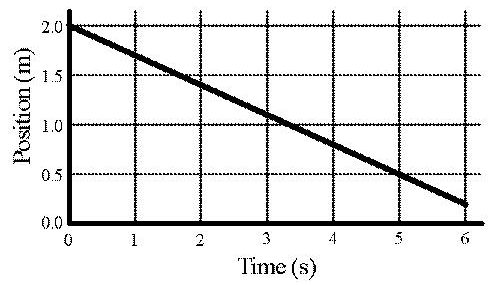# Problem: What is the x-component of the velocity (in m/s) for the object having the x(t) graph shown above?

###### FREE Expert Solution

X-component of velocity:

$\overline{){{\mathbf{v}}}_{{\mathbf{x}}}{\mathbf{=}}\frac{\mathbf{∆}\mathbf{x}\mathbf{\left(}\mathbf{t}\mathbf{\right)}}{\mathbf{∆}\mathbf{t}}}$

98% (132 ratings)###### Problem DetailsWhat is the x-component of the velocity (in m/s) for the object having the x(t) graph shown above?